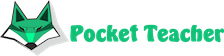# What are differential equations and how are they applied in practice

In many areas of science and technology, such as physics, biology, construction and even economics, real processes are described using mathematical functions or equations that determine the behavior of an object in depending on the change of various parameters (function arguments). If the equation includes not only the function itself, but also its derivatives, then it is called differential. The solution to such equations is often can be challenging enough. To make it easier, we provide the option to solve math and calculate differential equation online .

## Using Differential EquationsThe simplest example of using differential equations is finding the trajectory of a body a certain mass depending on external (gravity, friction) and internal (engine energy) impacts. That is, it is necessary to calculate its coordinates at any given time. However, from the impact of the applied force does not directly depend on the position of the body in space, but its acceleration motion is the second derivative. The result is a second-order differential equation. For in order to determine the coordinates of the body as a function of time, you need to solve this equation, or integrate it, as mathematicians say.

There are also much more complex processes that are described by functions that include derivatives more high orders or depending on many variables. There are situations for adequate reflection which require whole systems of differential equations. For their processing, powerful computers that use specially designed algorithms.

Tasks involving the solution of the simplest differential equations are included in the programs of schools and institutions. As a rule, it is necessary not only to find the correct answer, but also to describe the entire sequence operations and reasoning that led to the desired result. You can get the most detailed solution of many types of problems, equations and integrals online . This will provide pupils and students with significant assistance in the development of various sections. math and exam preparation.Our artificial intelligence solves complex math problems in seconds.

We will solve your exam, homework, olympiad problems with detailed steps. You will need just to copy the solution.